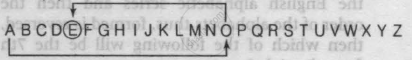# Which Letter Will Be 10th to the Left of the 15th Letter from the Left End of the Following Sequence? a B C D E F G H I J K L M N O P Q R S T U V W X Y Z - Logical Reasoning

MCQ

Which letter will be 10th to the left of the 15th letter from the left end of the following sequence?

A B C D E F G H I J K L M N O P Q R S T U V W X Y Z

• E

• F

• G

• H

#### Solution

E
Explanation:

10th to left15th from the left end
15th from the left end is O and 10th to the left of O is E.

Concept: Alphabet and Numeric Test
Is there an error in this question or solution?